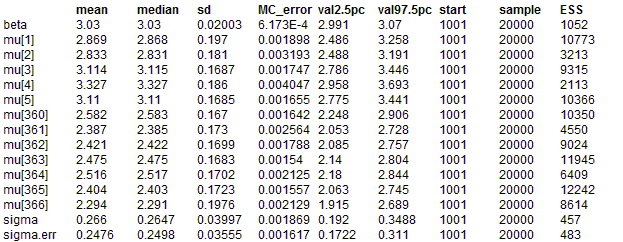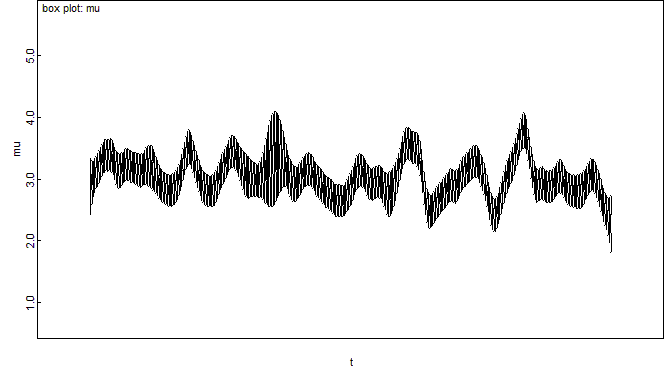#### Random walk priors for temporal smoothing of daily air pollution estimates

Shaddick and Wakefield (2002) consider spatiotemporal modelling of daily ambient air pollution at a number of monitoring sites in London. Here we take a subset of their data on a single pollutant measured at one site for 366 days, and model temporal autocorrelation using a random walk prior.

Conditional on the underlying mean concentration μt on day t, the likelihood for the observed pollution concetration Yt is assumed to be independent Normal i.e.

Yt ~ Normal(μt, τerr) where 1/τerr is the measurement error variance
μt = β + θt

where β is the overall mean pollution concentration at the site, and θt is a (zero mean) random error term representing daily fluctuations about this mean. To reflect the prior belief that these daily fluctuations are correlated, a random walk prior is assumed forθ = 1 , ......, θ366 } (see equation 7 in Shaddick and Wakefield):
θt | θ-t    ~ Normal ( θt+1, φ )   for t = 1
~ Normal ( (θt-1 + θt+1)/2, φ / 2 )   for t = 2, ...., T-1
~ Normal ( θt-1, φ )   for t = T

where θ-t denotes all elements of θexcept the θt. This prior may be specified in BUGS using the rand.walk distribution.

The RW(1) reflects prior beliefs about smoothness of first differences, i.e. sudden jumps between consecutive values of θ are unlikely. Alternatively, we may assume a second order random walk prior RW(2) for θ , which represents prior beliefs that the rate of change (gradient) of θ is smooth:
θt | θ-t ~ Normal ( 2θt+1 - θt+2, φ ) for t = 1
~ Normal ( (2θt-1 + 4θt+1 - θt+2) / 5, φ / 5 ) for t = 2
~ Normal ( (-θt-2 + 4θt-1 + 4θt+1 - θt+2) / 6, φ / 6 ) for t = 3, ...., T - 2
~ Normal ( (-θt-2 + 4θt-1 + 2θt+1) / 5, φ / 5 ) for t = T -1
~ Normal ( -θt-2+ 2θt-1, φ ) for t = T

Again this may be specified using the stoch.trend distribution in BUGS.
Model
The model code for fitting these two models is given below.
model {

#likelihood
for(t in 1:T) {
y[t] ~ dnorm(mu[t], tau.err)
mu[t] <- beta + theta[t]
}
theta[1:T] ~ rand.walk(tau)
#theta[1:T] ~ stoch.trend(tau)
beta ~ dflat()
# other priors
tau.err ~ dgamma(0.01, 0.01)      # measurement error precision
sigma.err <- 1 / sqrt(tau.err)
sigma2.err <- 1/tau.err
tau ~ dgamma(0.01, 0.01)            # random walk precision
sigma <- 1 / sqrt(tau)
sigma2 <- 1/tau
# include this variable to use in time series (model fit) plot
for(t in 1:T) { day[t] <- t }
}

Note that pollution concentrations were not measured every day. However it is necessary to include days with no measurements as missing values (NA) in the data set, otherwise the temporal neighbourhood structure cannot be specified correctly.
DataInits for chain 1    Inits for chain 2
Plus click on gen inits to generate initial values for the missing data
Results
RW(1) prior:Plot of posterior median (red line) and posterior 95% intervals (dashed blue lines) for mu[t] (the true mean daily pollutant concentration), with observed concentrations shown as black dots. (This plot was produced by selecting the model fit option from the Compare menu (available from the Inference menu), with mu specified as the node, day as the axis and y as other). Note that the dashed blue line shows the posterior 95% interval for the estimated mean daily concentration, and is not a predictive interval - hence we would not necessarily expect all of the observed data points to lie within the interval.

Equivalent plot assuming an RW(2) prior. Note the greater amount of smoothing imposed by this prior: